Next: 8. Outlook Up: 7. Simulation Results Previous: 7.4 Thermal Analysis of

# 7.5 Complete OpAmp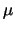A709

Thermal effects are of fundamental importance for the chip design of integrated circuits. Typical operational amplifiers (OpAmps) can deliver powers of 50-100 mW to a load, and as the output stage internally dissipates similar power levels the temperature of the chip rises in proportion to the dissipated output power [45,64]. As the transistors are very densely packed, self-heating of the output stage will affect all other transistors. This is especially true as silicon is a good thermal conductor, so the whole chip tends to rise to the same temperature as the output stage. However, small temperature gradients develop across the chip with the output stage being the heat source. The temperature coefficient of the junction voltage for forward-biased pn-junctions is known to be approximately -2 mV/K, that is to obtain the same current a smaller junction voltage is needed. As shown in Fig. 7.25 these temperature gradients appear across the input components of the OpAmp and induce an additional input voltage difference which is proportional to the output dissipated power.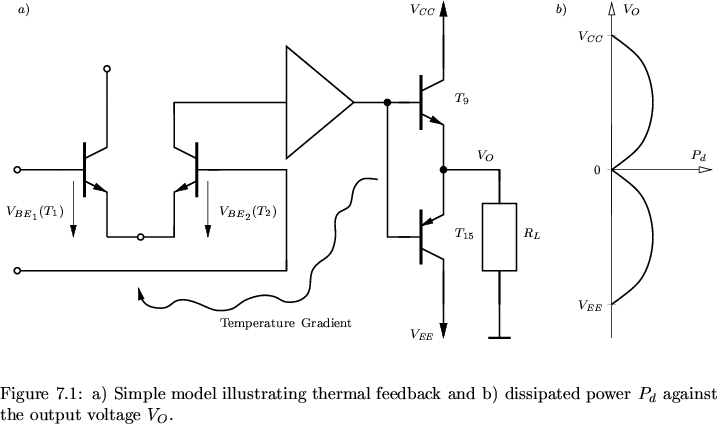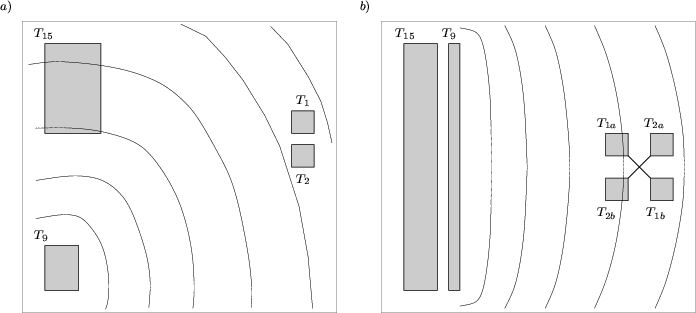To give an approximate expression for this effect, it can be assumed, to a first order, that the temperature difference T2 - T1 across a pair of matched and closely spaced components is given simply by

 T2 - T1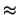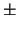KT . Pd (7.17)

with Pd being the power dissipated in the output stage, and KT a constant with dimension K/W. As the direction of the thermal gradient is unknown the plus/minus sign is necessary in (7.17). In fact, the sign may reverse polarity during the output swing as the dominant source of heat shifts from one transistor to the other. For the simple circuit model shown in Fig. 7.25a the thermally induced input voltage Vinth which adds to the input offset voltage can be approximated as
 Vinth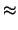KT . Pd . 2mV/K (7.18)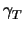. Pd (7.19)

For a thermally well designed OpAmp, typical values measured for KT are0.3 K/W and hence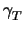0.6 mV/W. A good thermal layout is obtained when all coupled pairs are placed on thermal isolines. This is somehow difficult to obtain as the output transistors are geometrically separated and hence the isolines will be different depending on the state of the circuit [18,38]. A simple layout demonstrating this problem is shown in Fig. 7.26a whereas a configuration as shown in Fig. 7.26b can be used to minimize mismatch.

The dissipated power in the output stage of Fig. 7.25a can be written as

 Pd = (VS - VO) . IL =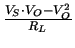(7.20)

with
 VS = VCC *1cmwhen *0.3cmVO > 0 (7.21) VS = VEE *1cmwhen *0.3cmVO < 0 (7.22)

A graph of Pd is shown in Fig. 7.25b with zero dissipation occurring at VO = 0, VCC, VEE and maximum dissipation occurring at VO = VCC/2 and VEE/2. It follows from (7.19) that Vinth has the same double-humped shape since it is just equal to a constant times Pd under DC conditions. However, the sign of Vinth may change during output swing in dependence of the layout. This is the case for the layout shown in Fig. 7.26a where the induced temperature difference changes sign when the output stage shifts operation from T9 to T15.

The completeA709 [59,25] as shown in Fig. 7.27 has been simulated considering thermal interaction between the input and the output stage. This circuit is of special interest as it is one of the SPICE benchmark circuits given in . The DC transfer characteristic has been calculated with and without thermal interaction. Consideration of thermal interaction was done by solving the lattice heat flow equation for the transistors T1, T2, T9 and T15 and by assuming a thermal network as shown in Fig. 7.28. The thermal conductances were assumed to be G1 = G2 = 2 mW/K and G9 = G15 = 10 mW/K while the coupling mismatch was modeled by G1, 9 = G1, 15 = Gk = 10 mW/K and G2, 9 = G2, 15 = Gk . (1 -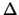) withbeing the mismatch parameter which is proportional to the temperature gradient across the input transistors .

The solution of the fully coupled equation system is possible with a proper iteration scheme. A small change in the output voltage during iteration causes a large change in the collector current of the conducting output transistor. The dissipated power changes and with it the temperature distribution inside the output transistor. This modified power alters the base-emitter voltages of the input transistors which produces a change in the base-emitter voltages of the output transistors. As all these coupling mechanisms are highly non-linear special precautions have to be taken. The iteration scheme given in Section 7.4 could be used. However, as the simulation failed very frequently for too large steps of the input voltage an additional failure criterion in the Thermal block was added. When the step of the input voltage was too large it caused oscillations in the solution which, due to the strong non-linearities, blew up the lattice temperatures. This took approximately 30 iterations which were very expensive in computational terms as each iteration took approximately 20 -200 seconds depending on the condition of the system matrix. So this event had to be detected as soon as possible. It was found that an abnormal behavior of the potential update norm E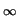(u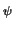) was a good indication of starting oscillations. Hence, whenever E(u) was larger than approximately 102 . VT after 10 iterations or whenever E(u) exceeded 105 . VT the iteration was canceled. Furthermore, the number of iterations was limited to 30.

An additional, even worse, phenomenon was observed when solving the fully coupled system in the Thermal block. Even for E(u) already as small as 10-2 the iterative solver failed and also generated a failure event.

These failure events were handled by reducing the increment of the input voltage by a factor of 2. Whenever the system could again be solved within 15 iterations the step size was doubled until the original step size was obtained.

The iteration scheme was inherited from ThermalDD as given in Section 7.4 with an unmodified Electric block. The ThermalPre and the Thermal block were augmented by the failure criterion given above

ThermalDD_2 : ThermalDD
{
aux f = !firstStep &&
((updateNormPot > 1e5) ||
(iterationCount > 10 && updateNormPot > 1e3) ||
(iterationCount > 30));

ThermalPre
{
failure = ^f;
}
Thermal
{
failure = ^f;
}
}


The DC transfer characteristic was calculated by stepping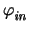from -1 mV to 1 mV with= 20V. From SPICE simulations the open-loop gain of theA709 was known to be approximately 35000 so for each step ofa step of 0.7 V could be expected for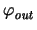which is quite large. However, no convergence problems occurred untilapproached 0 V. This was the most critical part of the simulation and several step reductions were necessary for both the pure electrical and the thermal simulation. The size of the system matrix was 37177 and 40449 for constant temperature and thermal simulation, respectively, and the simulation took 9 and 25 hours on a Linux Pentium II 350MHz workstation. For the thermal simulation the conditioning of the system matrix was found to be very poor and several step reductions were necessary.

The DC transfer characteristic is shown in Fig. 7.29 with the obvious humps resulting from thermal feedback effects. In Fig. 7.30 the open-loop voltage gain Av is shown and the dramatic impact of thermal coupling. The thermal conductances assumed in this simulation were very optimistic and an even stronger impact of thermal coupling has been published in literature [38,18]. For stronger coupling, even the sign of the open-loop voltage gain may change and cause the OpAmp to become unstable.

The maximum temperature and the contact temperature of the output stage are shown in Fig. 7.31. It is obvious that the self-heating inside the transistor plays only a minor role at these current levels. However, the power dissipated inside the device heats up the NPN transistor due to the resistive thermal boundary condition which obstruct the heat flow out of the transistor. This is in accordance to the commonly used assumption that the transistor can be modeled by a power source alone (cf. Appendix A). The PNP transistor has only a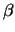of approximately 10 and comparable current levels have been obtained by increasing the emitter area of the transistor ( WPNP/WNPN = 5). Hence the locally generated heat density H is even smaller than for the NPN transistor and the temperature drop inside the device is negligible.

A similar situation occurs for input transistors T1 and T2. As they are biased with IC = 20A only self-heating is negligible and the contact temperature resembles the heat transfered from the output stage. As unsymmetric thermal conductivities have been assumed the temperature of T1 is always slightly higher than the temperature of T2. The temperature difference T2 - T1 is shown in Fig. 7.33 with a maximum of only -22 mK. Even this small temperature difference has a strong impact on the output characteristic due to the high gain of the circuit.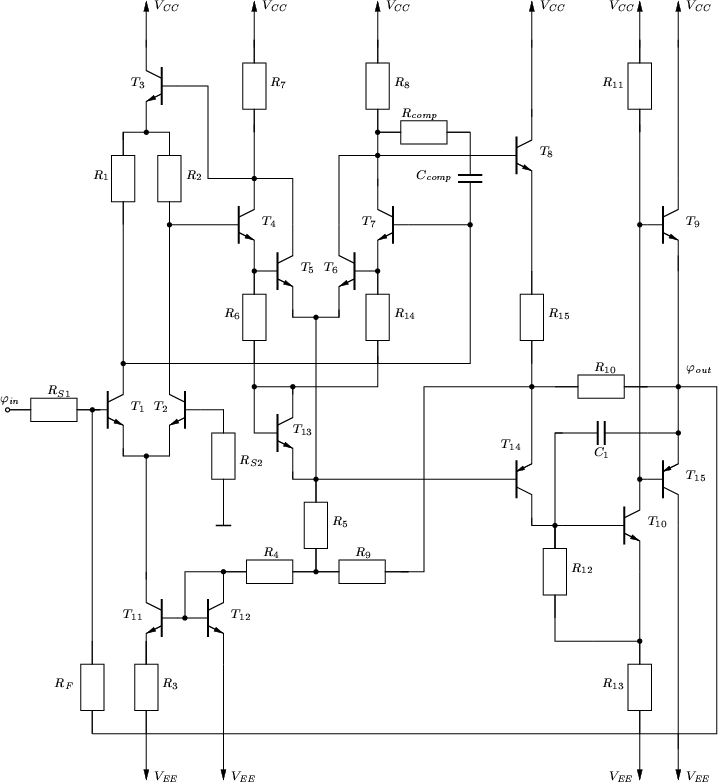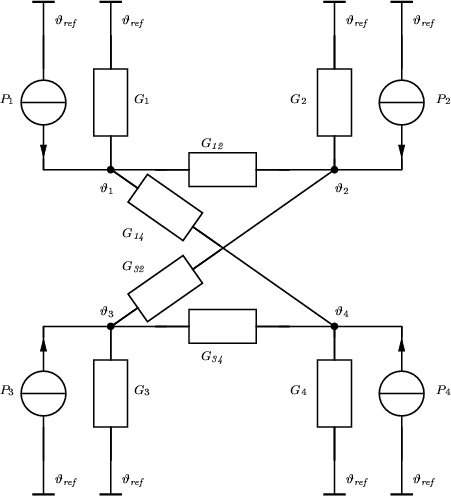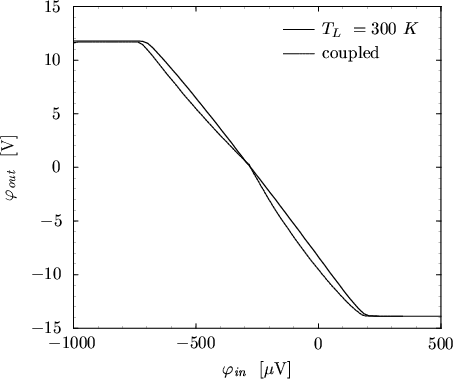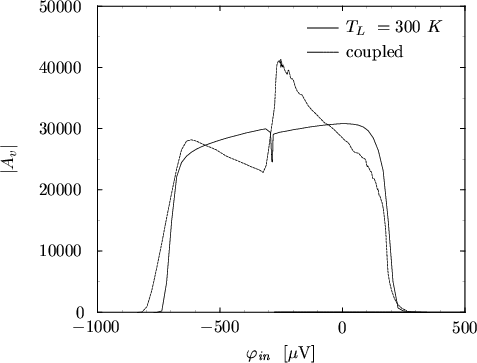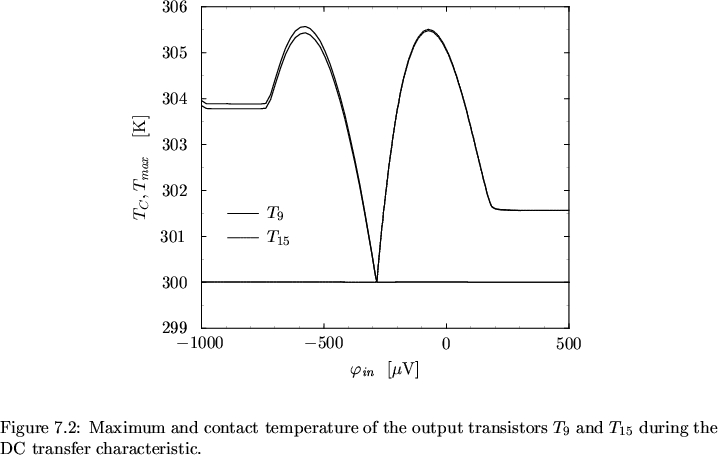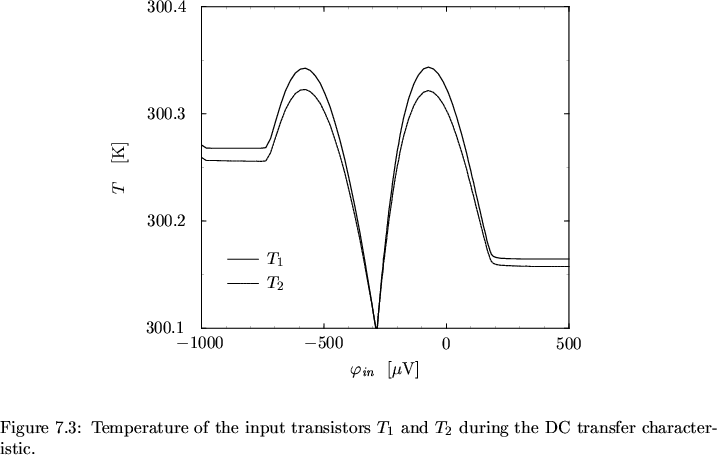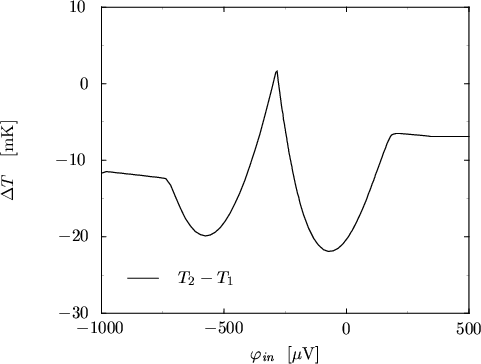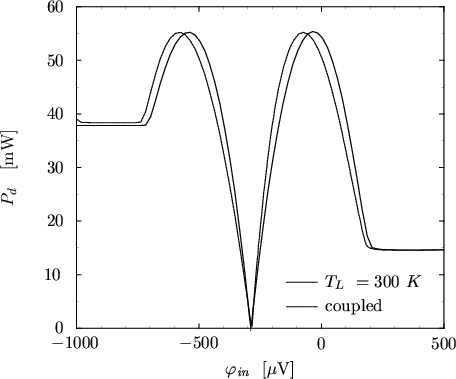Next: 8. Outlook Up: 7. Simulation Results Previous: 7.4 Thermal Analysis of
Tibor Grasser
1999-05-31